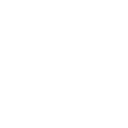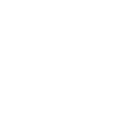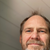0

# Limiting Function

Carl 6 years ago in IQANdesign updated by Ulrik Zakariasson (Software development) 6 years ago

Hi Guys,

In CodeySys we had a function where we could limit say the voltage input from a sensor etc.

So if we put it too 5% to 95% then no matter how higher or lower the input value was, they would stop at the limited values.

So if input was 100% the actual value out of the function was 95%, if it was 2% then the value out was 5%. is there a function in IQAN the same?

Cheers!!!+1

Use math channel (MAC) and there Vector, Qcode: "Result:= Vector(input,(100,95),(2,5))"